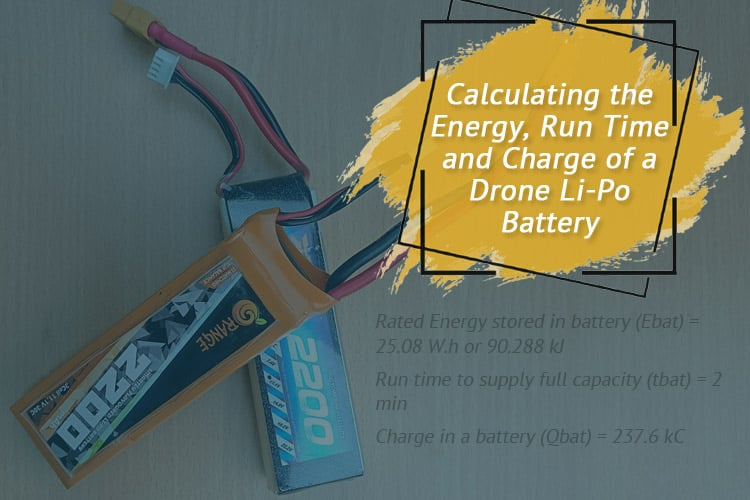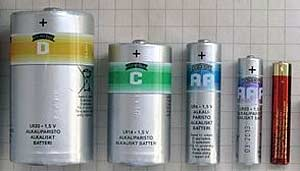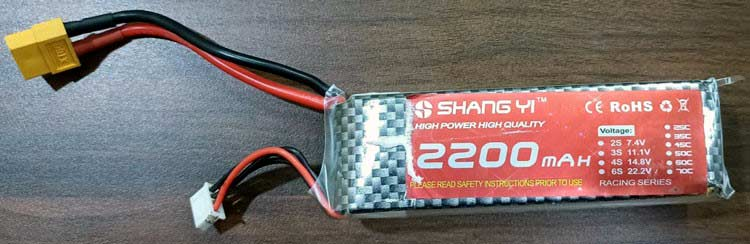# Calculating the Energy, Run Time and Charge of a Drone Li-Po Battery

Published  December 20, 2021   0The Lipo batteries are commonly used in drones and to power portable DIY projects that need to work for longer periods of time. These Lipo batteries are now utilized in drones due to their lightweight and high-power delivery capacity in a small package. In this article, we are going to calculate energy, run time, and charge calculations for the Lipo battery. But you can use this method of calculation for any type of battery. Here, throughout this tutorial, we will calculate the theoretical capacity, charge, stored energy, and run time of a Lipo battery.

### AAA, AA, C and D Batteries

The smallest form of an electric device capable of generating electrical energy from chemical processes is a dry cell, which consists of two electrodes, a chemical mixture, and a casing. It's a type of battery that's used to power small electronic gadgets like flashlights. Depending on its chemistry, a cell's nominal voltage ranges from 1 to 3 volts. AAA, AA, C, and D cells are some examples (batteries).Although all AAA, AA, C, and D batteries are rated at 1.5 volts, there is significant variability in their performance. Voltage and current are the two most crucial factors in every electrical circuit or device. Voltage can be compared to the water pressure that drives items down a pipe, while current can be compared to the amount of water flowing through the pipe. Some electrical gadgets require a lot of currents, but not a lot of voltage to operate. This is when the battery size comes into play. The D-size battery has a higher current output than the C, AA, and AAA size batteries. So, even though both AA and AAA batteries are rated at 1.5 volts, the AA battery will deliver greater current than the AAA battery.

### Calculation of Rated Energy, Run Time, and Charge Stored in Lipo Battery (12v, 2200mAh, 30C)

Before we go through how to calculate, let's go over some definitions which are required due to the usage of ambiguous terminology in the field of electric batteries. A battery is an electrochemical device made up of one (single-cell battery) or multiple (multi-cell battery) electrochemical cells installed in a single casing and connected in series and parallel to power various electrical devices.What is Crate in Battery?

Crate (or Crating) is a dimensionless value that is defined as the discharge current divided by the theoretical current draw at which the battery would provide its rated capacity in one hour. For a battery with a rated capacity Cbat = 8 Ah, a 2C discharge with current Ibat=16 A would supply the power for 0.5 hours. In one hour, a 1C drain of the same battery would deliver the specified capacity with an 8 A current. Despite the fact that Cbat is in ampere-hours and Ibat is in amperes, Crate is a dimensionless value. It's also worth noting that if the battery is depleted at a greater Crate, it will offer less energy.

What is the Depth of Discharge of Battery?

Frequently, the battery's full capacity cannot be used without causing damage to the battery. The permissible depth of discharge (DOD) of a battery, as mentioned in its specifications, regulates the percentage of energy that can be extracted from the battery. Lead-acid batteries are used to start automobile engines and are not designed for the deep drain. Some batteries can only be discharged to 30% capacity, which means that only 30% of their capacity may be used to power the load.

The relationship between the current drawn from the battery, its capacity, and C-rate is shown in the following formula:

`Ibat = Crate * Cbat`

Where

Ibat is the current drawn from the battery in amperes.

Cbat is the battery's rated capacity in amperes-hours (amperes times hours), which is normally printed on the battery.

Calculating the Crate

The battery Crate is calculated by dividing the discharge current by the theoretical current draw at which the battery would supply its rated capacity in one hour.

For 2200mAh, 30C battery

`Ibat= 2200 * 30 = 66A`

The Crate and the run time t are inversely related.

```t = 1 / Crate, or
Crate = 1 / t
t = 1 / 30 = 0.333 per min
tbat = 0.333 * 60 = 2 min```

It should be noted that this is a theoretical run time. The actual run time will be roughly 30% less than that calculated. It's also worth noting that the battery's allowable depth of discharge (DOD) restricts its operational time.

Calculating the Rated Energy Stored in Battery

The following formula is used to calculate the Rated Energy in watt-hours contained in a battery:

```Ebat= Vbat* Cbat
Ebat = 11.4V * 2200mAh = 25.08 W h```

Where

Ebat is the watt-hours of rated energy stored in the battery.,

Vbat is the battery's rated voltage in volts, and

Cbat is the battery's rated capacity in Ah.

Calculating the Energy

The following formula is used to determine the energy in joules (watts-seconds):

```Ebat.jouls = 3600 * Ebat,W h
Ebat.jouls = 3600 * 342 = 90.288 kJ```

We know that one ampere flowing in a wire for one second will use 1 coulomb of charge. Therefore, the charge in the battery is defined from Q = I · t.

```Qbat= 3600 * Ibat
Qbat= 3600 * 66 = 237.6 kC                                                               ```

Where

Qbat is the charge in a battery which is measured in coulombs (C) and Ibat is the current drawn from the battery in amperes.

### Conclusion

So, for 12V, 2200mAH, and 30C Lipo batteries,

```Rated Energy stored in battery (Ebat) = 25.08 W.h or 90.288 kJ

Run time to supply full capacity (tbat) = 2 min

Charge in a battery (Qbat) = 237.6 kC```
Tags